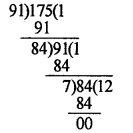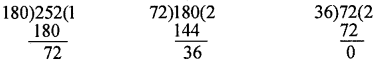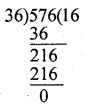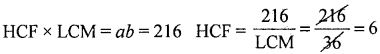# 1st PUC Basic Maths Question Bank Chapter 1 Number Theory

Students can Download Basic Maths Chapter 1 Number Theory Questions and Answers, Notes Pdf, 1st PUC Basic Maths Question Bank with Answers helps you to revise the complete Karnataka State Board Syllabus and score more marks in your examinations.

## Karnataka 1st PUC Basic Maths Question Bank Chapter 1 Number Theory

Question 1.
Find the GCD of 1819 and 3587The GCD of 1819 and 3587 is 17
⇒ (1819,3587) = 17.

Question 2.
Find GCD of 91 and 175 and write is the form 91x + 175y.
84 = 175 – 91(1) ………. (1)
7 =91 – 84(1) ……….. (2)
∴ (91, 175) = 7Question 3.
Find number of positive divisions and the sum of the any five division of 4896.
4896 = 25 × 32 × 171
Tn = (1 + L1)(1 + L2)(1 + L3)
= (1 + 5)(1 + 2)(1 + 1) = 6.3 .2 = 36Question 4.
Find the HCF of 180, 252, and 576
HCF of 180 and 252∴ HCF of 180 and 252 is 36

Now find HCF of 36 and 576∴ HCF of 36 and 576 is 36
∴ ACP of 180,252 and 576 is 36Question 5.
Find the greatest number which divides 989 and 1327 learn remainder 5 and 7 respectively.